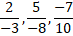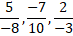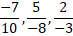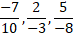# Quiz Discussion

The rational number for the recurring decimal 0.125125..... is :

Course Name: Quantitative Aptitude

• 1]

63/487

• 2]

119/993

• 3]

125/999

• 4]

None of these

##### Solution
No Solution Present Yet

#### Top 5 Similar Quiz - Based On AI&ML

Quiz Recommendation System API Link - https://fresherbell-quiz-api.herokuapp.com/fresherbell_quiz_api

# Quiz
1
Discuss

If 2994 ÷ 14.5 = 172, then 29.94 ÷ 1.45 = ?

• 1] 0.172
• 2] 1.72
• 3] 17.2
• 4] 172
##### Solution
2
Discuss

$$3.\overline {87} - 2.\overline {59} = ?$$

• 1]

1.20

• 2]

$$1.\overline 2$$

• 3]

$$1.\overline {27}$$

• 4]

$$1.\overline {28}$$

##### Solution
3
Discuss

Which of the following has fractions in ascending order ?

• 1]

$$\frac{2}{3},\frac{3}{5},\frac{7}{9},\frac{9}{{11}},\frac{8}{9}$$

• 2]

$$\frac{3}{5},\frac{2}{3},\frac{9}{11},\frac{7}{{9}},\frac{8}{9}$$

• 3]

$$\frac{3}{5},\frac{2}{3},\frac{7}{9},\frac{9}{{11}},\frac{8}{9}$$

• 4]

$$\frac{8}{9},\frac{9}{11},\frac{7}{9},\frac{2}{{3}},\frac{3}{5}$$

• 5]

$$\frac{8}{9},\frac{9}{11},\frac{7}{9},\frac{3}{{5}},\frac{2}{3}$$

##### Solution
4
Discuss

The value of $$\frac{{3.157 \times 4126 \times 3.198}}{{63.972 \times 2835.121}}$$    is closest to :

• 1] 0.002
• 2] 0.02
• 3] 0.2
• 4] 2
##### Solution
5
Discuss

383 × 38 × 3.8 = ?

• 1] 55305.2
• 2] 56305.4
• 3] 57305.6
• 4] 58305.8
• 5] None of these
##### Solution
6
Discuss

The expression (11.98 × 11.98 + 11.98 × X + 0.02 × 0.02) will be a perfect square for X equal to:

• 1] 0.02
• 2] 0.2
• 3] 0.04
• 4] 0.4
##### Solution
7
Discuss

The price of commodity X increases by 40 paise every year, while the price of commodity Y increases by 15 paise every year. If in 2001, the price of commodity X was Rs. 4.20 and that of Y was Rs. 6.30, in which year commodity X will cost 40 paise more than the commodity Y ?

• 1]

2010

• 2]

2011

• 3]

2012

• 4]

2013

##### Solution
8
Discuss

Which of the following fractions is greater than 3/4 and less than 5/6 ?

• 1]

1/2

• 2]

2/3

• 3]

4/5

• 4]

9/10

##### Solution
9
Discuss

The arrangement of rational numbers, -7/10, 5/-8, 2/-3  in ascending order is :

• 1]• 2]• 3]• 4]##### Solution
10
Discuss

The value of $$\frac{{489.1375 \times 0.0483 \times 1.956}}{{0.0873 \times 92.581 \times 99.749}}$$     is closest to:

• 1] 0.006
• 2] 0.06
• 3] 0.6
• 4] 6
# Quiz